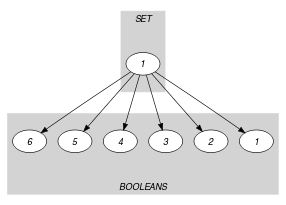Origin
Constraint

$\mathrm{𝚕𝚒𝚗𝚔}_\mathrm{𝚜𝚎𝚝}_\mathrm{𝚝𝚘}_\mathrm{𝚋𝚘𝚘𝚕𝚎𝚊𝚗𝚜}\left(\mathrm{𝚂𝚅𝙰𝚁},\mathrm{𝙱𝙾𝙾𝙻𝙴𝙰𝙽𝚂}\right)$

Arguments
 $\mathrm{𝚂𝚅𝙰𝚁}$ $\mathrm{𝚜𝚟𝚊𝚛}$ $\mathrm{𝙱𝙾𝙾𝙻𝙴𝙰𝙽𝚂}$ $\mathrm{𝚌𝚘𝚕𝚕𝚎𝚌𝚝𝚒𝚘𝚗}\left(\mathrm{𝚋𝚘𝚘𝚕}-\mathrm{𝚍𝚟𝚊𝚛},\mathrm{𝚟𝚊𝚕}-\mathrm{𝚒𝚗𝚝}\right)$
Restrictions
 $\mathrm{𝚛𝚎𝚚𝚞𝚒𝚛𝚎𝚍}$$\left(\mathrm{𝙱𝙾𝙾𝙻𝙴𝙰𝙽𝚂},\left[\mathrm{𝚋𝚘𝚘𝚕},\mathrm{𝚟𝚊𝚕}\right]\right)$ $\mathrm{𝙱𝙾𝙾𝙻𝙴𝙰𝙽𝚂}.\mathrm{𝚋𝚘𝚘𝚕}\ge 0$ $\mathrm{𝙱𝙾𝙾𝙻𝙴𝙰𝙽𝚂}.\mathrm{𝚋𝚘𝚘𝚕}\le 1$ $\mathrm{𝚍𝚒𝚜𝚝𝚒𝚗𝚌𝚝}$$\left(\mathrm{𝙱𝙾𝙾𝙻𝙴𝙰𝙽𝚂},\mathrm{𝚟𝚊𝚕}\right)$
Purpose

Make the link between a set variable $\mathrm{𝚂𝚅𝙰𝚁}$ and those 0-1 variables that are associated with each potential value belonging to $\mathrm{𝚂𝚅𝙰𝚁}$: The 0-1 variables, which are associated with a value belonging to the set variable $\mathrm{𝚂𝚅𝙰𝚁}$, are equal to 1, while the remaining 0-1 variables are all equal to 0.

Example
$\left(\begin{array}{c}\left\{1,3,4\right\},\hfill \\ 〈\begin{array}{cc}\mathrm{𝚋𝚘𝚘𝚕}-0\hfill & \mathrm{𝚟𝚊𝚕}-0,\hfill \\ \mathrm{𝚋𝚘𝚘𝚕}-1\hfill & \mathrm{𝚟𝚊𝚕}-1,\hfill \\ \mathrm{𝚋𝚘𝚘𝚕}-0\hfill & \mathrm{𝚟𝚊𝚕}-2,\hfill \\ \mathrm{𝚋𝚘𝚘𝚕}-1\hfill & \mathrm{𝚟𝚊𝚕}-3,\hfill \\ \mathrm{𝚋𝚘𝚘𝚕}-1\hfill & \mathrm{𝚟𝚊𝚕}-4,\hfill \\ \mathrm{𝚋𝚘𝚘𝚕}-0\hfill & \mathrm{𝚟𝚊𝚕}-5\hfill \end{array}〉\hfill \end{array}\right)$

In the example, the 0-1 variables associated with the values 1, 3 and 4 are all set to 1, while the other 0-1 variables are set to 0. Consequently, the $\mathrm{𝚕𝚒𝚗𝚔}_\mathrm{𝚜𝚎𝚝}_\mathrm{𝚝𝚘}_\mathrm{𝚋𝚘𝚘𝚕𝚎𝚊𝚗𝚜}$ constraint holds since its first argument $\mathrm{𝚂𝚅𝙰𝚁}$ is set to $\left\{1,3,4\right\}$.

Typical
 $|\mathrm{𝙱𝙾𝙾𝙻𝙴𝙰𝙽𝚂}|>1$ $\mathrm{𝚛𝚊𝚗𝚐𝚎}$$\left(\mathrm{𝙱𝙾𝙾𝙻𝙴𝙰𝙽𝚂}.\mathrm{𝚋𝚘𝚘𝚕}\right)>1$
Symmetry

Items of $\mathrm{𝙱𝙾𝙾𝙻𝙴𝙰𝙽𝚂}$ are permutable.

Usage

This constraint is used in order to make the link between a formulation using set variables and a formulation based on linear programming.

Systems
Keywords
Derived Collection
$\mathrm{𝚌𝚘𝚕}\left(\begin{array}{c}\mathrm{𝚂𝙴𝚃}-\mathrm{𝚌𝚘𝚕𝚕𝚎𝚌𝚝𝚒𝚘𝚗}\left(\mathrm{𝚘𝚗𝚎}-\mathrm{𝚒𝚗𝚝},\mathrm{𝚜𝚎𝚝𝚟𝚊𝚛}-\mathrm{𝚜𝚟𝚊𝚛}\right),\hfill \\ \mathrm{𝚒𝚝𝚎𝚖}\left(\mathrm{𝚘𝚗𝚎}-1,\mathrm{𝚜𝚎𝚝𝚟𝚊𝚛}-\mathrm{𝚂𝚅𝙰𝚁}\right)\right]\hfill \end{array}\right)$
Arc input(s)

$\mathrm{𝚂𝙴𝚃}$ $\mathrm{𝙱𝙾𝙾𝙻𝙴𝙰𝙽𝚂}$

Arc generator
$\mathrm{𝑃𝑅𝑂𝐷𝑈𝐶𝑇}$$↦\mathrm{𝚌𝚘𝚕𝚕𝚎𝚌𝚝𝚒𝚘𝚗}\left(\mathrm{𝚜𝚎𝚝},\mathrm{𝚋𝚘𝚘𝚕𝚎𝚊𝚗𝚜}\right)$

Arc arity
Arc constraint(s)
$\mathrm{𝚋𝚘𝚘𝚕𝚎𝚊𝚗𝚜}.\mathrm{𝚋𝚘𝚘𝚕}=\mathrm{𝚜𝚎𝚝}.\mathrm{𝚘𝚗𝚎}⇔$$\mathrm{𝚒𝚗}_\mathrm{𝚜𝚎𝚝}$$\left(\mathrm{𝚋𝚘𝚘𝚕𝚎𝚊𝚗𝚜}.\mathrm{𝚟𝚊𝚕},\mathrm{𝚜𝚎𝚝}.\mathrm{𝚜𝚎𝚝𝚟𝚊𝚛}\right)$
Graph property(ies)
$\mathrm{𝐍𝐀𝐑𝐂}$$=|\mathrm{𝙱𝙾𝙾𝙻𝙴𝙰𝙽𝚂}|$

Graph model

The $\mathrm{𝚕𝚒𝚗𝚔}_\mathrm{𝚜𝚎𝚝}_\mathrm{𝚝𝚘}_\mathrm{𝚋𝚘𝚘𝚕𝚎𝚊𝚗𝚜}$ constraint is modelled with the following bipartite graph. The first set of vertices corresponds to a single vertex containing the set variable. The second class of vertices contains one vertex for each item of the collection $\mathrm{𝙱𝙾𝙾𝙻𝙴𝙰𝙽𝚂}$. The arc constraint between the set variable $\mathrm{𝚂𝚅𝙰𝚁}$ and one potential value $v$ of the set variable expresses the following:

• If the 0-1 variable associated with $v$ is equal to 1 then $v$ should belong to $\mathrm{𝚂𝚅𝙰𝚁}$.

• Otherwise if the 0-1 variable associated with $v$ is equal to 0 then $v$ should not belong to $\mathrm{𝚂𝚅𝙰𝚁}$.

Since all arc constraints should hold the final graph contains exactly $|\mathrm{𝙱𝙾𝙾𝙻𝙴𝙰𝙽𝚂}|$ arcs.

Parts (A) and (B) of Figure 5.234.1 respectively show the initial and final graph associated with the Example slot. Since we use the $\mathrm{𝐍𝐀𝐑𝐂}$ graph property, the arcs of the final graph are stressed in bold. The $\mathrm{𝚕𝚒𝚗𝚔}_\mathrm{𝚜𝚎𝚝}_\mathrm{𝚝𝚘}_\mathrm{𝚋𝚘𝚘𝚕𝚎𝚊𝚗𝚜}$ constraint holds since the final graph contains exactly 6 arcs (one for each 0-1 variable).

##### Figure 5.234.1. Initial and final graph of the $\mathrm{𝚕𝚒𝚗𝚔}_\mathrm{𝚜𝚎𝚝}_\mathrm{𝚝𝚘}_\mathrm{𝚋𝚘𝚘𝚕𝚎𝚊𝚗𝚜}$ constraint(a)(b)
Signature

Since the initial graph contains $|\mathrm{𝙱𝙾𝙾𝙻𝙴𝙰𝙽𝚂}|$ arcs the maximum number of arcs of the final graph is equal to $|\mathrm{𝙱𝙾𝙾𝙻𝙴𝙰𝙽𝚂}|$. Therefore we can rewrite the graph property $\mathrm{𝐍𝐀𝐑𝐂}=|\mathrm{𝙱𝙾𝙾𝙻𝙴𝙰𝙽𝚂}|$ to $\mathrm{𝐍𝐀𝐑𝐂}\ge |\mathrm{𝙱𝙾𝙾𝙻𝙴𝙰𝙽𝚂}|$ and simplify $\underline{\overline{\mathrm{𝐍𝐀𝐑𝐂}}}$ to $\overline{\mathrm{𝐍𝐀𝐑𝐂}}$.